International Journal of Energy and Power Engineering
Volume 4, Issue 3, June 2015, Pages: 146-152

PMSG Based Wind Energy Conversion with Space Vector Modulation

M. Magesh Kumar1, R. Sundareswaran2

1ME power system engineering, Chandy college of engineering, Tuticorin, India

2AP/EEE Department, Chandy college of engineering, Tuticorin, India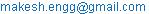(M. M. Kumar)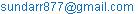(R. Sundareswaran)

M. Magesh Kumar, R. Sundareswaran. PMSG Based Wind Energy Conversion with Space Vector Modulation. International Journal of Energy and Power Engineering. Vol. 4, No. 3, 2015, pp. 146-152. doi: 10.11648/j.ijepe.20150403.13

Abstract: Space Vector Modulation (SVM) based Direct Torque Control (DTC) scheme is used for a variable –speed direct drive Permanent Magnet Synchronous Generator (PMSG) in a wind power generation system. SVM Provides more flexibility for inverter voltage utilization, in order to compensate the torque and flux errors in a smoother way than conventional DTC. SVM based DTC maintains constant switching frequency and it can be applied using closed loop torque control, for minimization of torque ripple. Over the conventional Doubly Fed Induction Generator (DFIG), PMSG has several advantages. The PMSG based on Wind Energy Conversion System (WECS) can be connected to the turbine without gearbox. The gearbox causes in the cost of maintenance, and then it will decrease the weight of the nacelle. Additionally, the generators have a better efficiency and have been slightly cheaper. This provides maximum flexibility, enabling full real and reactive power control. The SVM-DTC regulated PMSG wind turbine can achieve fast torque response, relatively low torque ripples, and extracts the maximum power. The effectiveness of the SVM-DTC control scheme for PMSG has been demonstrated by MATLAB simulation.

Keywords: Space Vector Modulation, Direct Torque Control, Permanent Magnet Synchronous Generator, Doubly Fed Induction Generator, Wind Energy Conversion Systems

1. Introduction

The issue of global warming has been raised due to the consumption of oil, coal and natural resource for the electricity producing process. Apart from that, the amounts of these fuels on earth are now decreasing day by day. Thus, the focus has been shifted to the green alternative energy which is not polluted and has no impact on the environment. The power of wind is now being explored which the researcher believes that it has all the qualifications to replace traditional fuel since it has less effect to global warming. During the past decade, the amounts of wind capacity have been installed every three years. Around 83% of wind capacities are located in these five countries, German, United States of America, Denmark, India and Spain .

Wind energy conversion system (WECS) consist of wind turbine, pitch angle control, drive train, generator and power converter . Fig. 1 shows the typical Wind Energy Conversion System.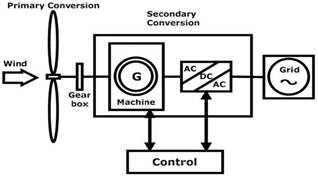Fig. 1. Typical Wind Energy Conversion System.

There are various kinds of generators used in WECS such as induction generator (IG), doubly fed induction generator (DFIG) and permanent magnet synchronous generator (PMSG) . The PMSG based on WECS can connect to the turbine without using gearbox, which significantly reduces the construction, operation, and maintenance costs, and then it will decrease the weight of nacelle . These benefits have made the direct drive PMSG-based WTGs the most popular configuration in multi-MW offshore applications . In the last few decades, a direct-torque control (DTC) method was proposed  and naturally introduced to the permanent-magnet synchronous motor (PMSM) drive systems , . In the DTC, the inverter and the machine are conceived as a whole so that the stator voltage vectors can be selected directly according to the differences between the reference and actual torques and stator flux linkages. This method not only removes the need for complicated computation of reference frame transformation and high dependence of the decoupled current control on machine parameters but also achieves a faster torque response.

2. Direct Drive PMSG

The most important difference between the geared generator and the direct-drive generator is the rotation speed of the generator. The direct-drive generator rotates at low speed, because the rotor of generator is directly connected on the hub of rotor blades. This low speed makes it necessary to produce a very high rated torque in the direct-drive generator, since the size of this generator depends on the rated torque rather than the rated power. The direct-drive generator mostly used in the market can be classified to two concepts. They are (i) electrically excited synchronous generator (EESG) and (ii) permanent magnet synchronous generator (PMSG)  .

The advantages of the direct-drive generator compared to the geared generator are Simplified drive train by omitting the gearbox, high overall efficiency and reliability, high availability, low noise of the drive train.

A direct-drive generator is usually heavier than a geared generator because it has to make a high rated torque as mentioned above. To increase the efficiency, to reduce the weight of the active parts, and to keep the end winding losses small, the direct-drive generator is usually designed with a large diameter and small pole pitch. Fig 2.Shows Direct Drive PMSG for wind energy conversion system.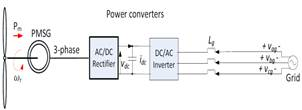Fig. 2. Direct Drive Pmsg Wecs.

In the considerations of the energy yield and reliability, direct-drive systems seem to be more powerful than the geared systems, especially for offshore. Direct-drive systems, which are operated in low speed, also have disadvantages as the large diameter, large weight, and high cost compared to geared systems.

3. Direct-Drive Pmsg Wind Turbine System Modelling

The configuration of a direct-drive PMSG wind turbine is connected to the PMSG directly . The electrical power generated by the PMSG is transmitted to a power grid and/or supplied to a load via a variable-frequency converter, which consists of a machine-side converter (MSC) and a grid-side converter connected back-to-back via a dc link .

A. Wind turbine aerodynamic model

The mechanical power that a wind turbine extracts from wind is given by ,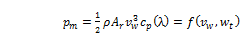(1)

Where,

ρ is the air density

Ar is the area swept by the blades

vω is the wind speed

CP is the turbine power coefficient

ωt is the turbine shaft or generator rotor speed

λ is the tip-speed ratio.

Tip-speed ratio is defined by ,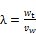R                             (2)

Where, R is the radius of the wind turbine rotor plane.

B. Modeling of the PMSG

The dynamic equations of a three-phase PMSG can be written in a synchronously rotating dq reference frame as,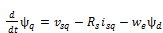(3)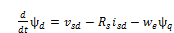(4)

Where,

vsq and vsd are the q- and d-axis stator terminal voltages, respectively,

isq and isd are the q- and d-axis stator currents, respectively,

Rs is the resistance of the stator windings,

ωe is the electrical angular velocity of the rotor and,

ψq and ψd are the q- and d-axis flux linkages of the PMSG, respectively.

q-axis and d-axis flux linkages ψq and ψd of the PMSG are given by,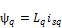(5)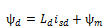(6)

Where,

Lq and Ld are the q- and d-axis inductances of the PMSG, respectively and,

ψm is the flux linkage generated by the permanent magnets.

The electromagnetic torque Te can be calculated by,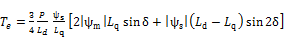(7)

Where,

p is the number of pole pairs,

s | and |ψm | are the magnitudes of the stator and rotor flux linkages, respectively,

δ is called the torque angle.

For a nonsalient-pole PMSG, Ld = Lq = Ls                    (8)

Then, the electromagnetic torque can be simplified as,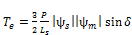(9)

C. Modeling of the shaft system

As the wind turbine is connected to the PMSG directly, the shaft system of the WTG can be represented by a two-mass model . The motion equation is then given by,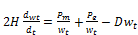(10)

Where,

2H is the total inertia constant of the WTG, and D is the damping coefficient.

4. Space Vector PWM Technique

Space vector PWM refers to a special switching scheme of the six power semiconductor switches of a three phase power converter . Space vector PWM (SVPWM) has become a popular PWM technique for three-phase voltage-source inverters in applications such as control of induction and permanent magnet synchronous motors. The drawbacks of the sinusoidal PWM and hysteresis-band current control are reduced using this technique. Instead of using a separate modulator for each of the three phases (as in the previous techniques), the complex reference voltage vectors processed as a whole. Therefore, the interaction between the three motor phases is considered. It has been shown, that SVPWM generates less harmonic distortion in both output voltage and current applied to the phases of an ac motor and provides a more efficient use of the supply voltage in comparison with sinusoidal modulation techniques. SVPWM provides a constant switching frequency and therefore the switching frequency can be adjusted easily. Although SVPWM is more complicated than sinusoidal PWM and hysteresis band current control, it may be implemented easily with modern DSP based control systems.

A.  Principle of Space Vector PWM

Eight possible combinations of on and off patterns may be achieved. The on and off states of the lower switches are the inverted states of the upper ones . The phase voltages corresponding to the eight combinations of switching patterns can be calculated and then converted into the stator two phase (αβ) reference frames. This transformation results in six non-zero voltage vectors and two zero vectors. The non-zero vectors form the axes of a hexagon containing six sectors (V1 − V6) as shown in Fig. 3.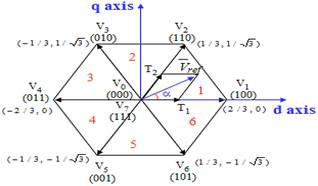Fig. 3. Non-zero vectors forming a hexagon and zero vectors.

The angle between any adjacent two non-zero vectors is 60 electrical degrees. The zero vectors are at the origin and apply a zero voltage vector to the motor. The envelope of the hexagon formed by the non-zero vectors is the locus of the maximum output voltage .

The maximum output phase voltage and line-to-line voltage that can be achieved by applying SVPWM are: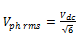(11)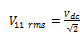(12)

Therefore the dc voltage Vdc for a given motor R.M.S. voltage Vphrms is,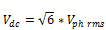(13)

Practically, only two adjacent non-zero voltage vectors Vx and Vx+60 and the zero vectors should be used. Depending on the reference voltages Vα and Vβ, the corresponding sector is firstly determined. The sector identification is carried out using the flow chart in Fig.4.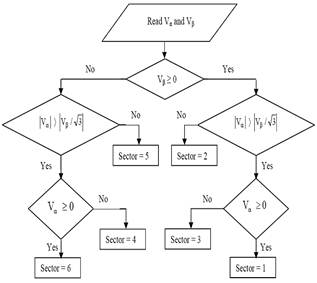Fig. 4. Flow chart for determining the sector.

B.  Comparison of Sine PWM and Space Vector PWM

·     SVPWM generates less harmonic distortion in both output voltage and current.

·     Provides a more efficient use of the supply voltage in comparison with sinusoidal modulation techniques.

·     SVPWM provides a constant switching frequency and therefore it can be adjusted easily.

·     SVPWM is more complicated than sinusoidal PWM and hysteresis band current control.

·     It may be implemented easily with modern DSP based control systems.

Fig. 5 shows that the Voltage Utilization of SVPWM is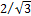times more than Sine PWM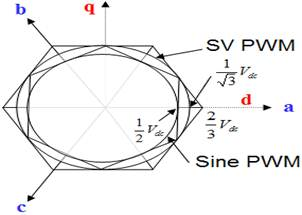Fig. 5. Voltage Utilization of SVPWM and SinePWM.

C.  Realization of Space Vector PWM

·     Step 1. Determine Vd, Vq, Vref, and angle (a).

·     Step 2. Determine time duration T1, T2, T0.

·     Step 3. Determine the switching time of each transistor (S1 to S6).

5. Space Vector Modulation-Direct Torque Control

In this new method disadvantages of the classical DTC are eliminated. Basically, the DTC-SVM strategies are the methods, which operate with constant switching Frequency. The DTC–SVM scheme combines advantages of FOC system (constant switching frequency, SVM and PI controllers) and DTC algorithm (simple structure without current control loops and coordinate transformation, direct torque and flux control) and recently has been successfully used in industrial drives.

Direct flux and torque control with space vector modulation (DTC-SVM) schemes are proposed in order to improve the classical DTC. The DTC-SVM strategies operate at a constant switching frequency. In the control structures, space vector modulation (SVM) algorithm is used. The type of DTC-SVM strategy depends on the applied flux and torque control algorithm. Basically, the controllers calculate the required stator voltage vector and then it is realized by space vector modulation technique.

A.  DTC–SVM Principle

DTC–SVM can be implemented in various ways [6, 7, 8, and 10]. The Fig.6 shows that the DTC-SVM structure. This control scheme does not have any differentional algorithms, like in  or . Instead of this in parallel structure of DTC–SVM reference voltage for space vector modulator is calculated by two linear PI regulators as two adjacent stator voltage components Usx and Usy. Usx is calculated by stator flux PI controller, whereas Usy is calculated by electromagnetic torque PI controller. Additionally, for high speed region, where stator flux magnitude has to be lowered, in parallel DTC–SVM structure field weakening algorithm can be applied easily.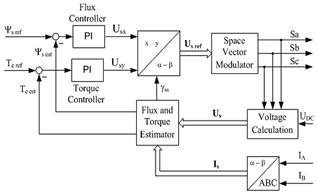Fig. 6. DTC–SVM structure.

In DTC–SVM torque and flux are controlled directly. In traction torque control loop is used for speed regulation. Also field weakening algorithms are necessary for induction induction motor based traction drives. Therefore, presented algorithm is suitable for applications like: trams, trolleybuses, underground or fast city train.

B.  SVPWM- DTC Modelling

The schematic of the proposed SVM-DTC for a nonsalient pole PMSG WTG is shown in Fig.7. ψsαβ = [ ψ, ψ]T and usαβ = [u, u]T are the estimated stator flux linkage vector and the resultant stator voltage space vector in the stationary αβ reference frame, respectively and θre and ωt are the estimated electrical angular rotor position and rotor speed, respectively.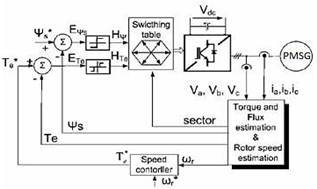Fig. 7. SVM-DTC for PMSG.

SVM-DTC scheme retains the advantages of the conventional DTC, such as no coordinate transformation, no current control, etc. However, instead of adopting a switching table and hysteresis comparators, a reference voltage vector calculator (RVVC) is designed to determine the desired voltage vector u αβ. The detailed analysis is given below.

The reference torque angle of this system in a discrete time form can be written as,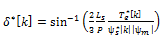(14)

The reference stator flux angle θs[k] can be obtained from the following equation: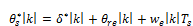(15)

Where the effect of the rotor speed is taken into consideration by adding the term ωe[k]Ts to compensate for the rotor position increment when the PMSG operates at a high speed. It is well known that the stator voltages of an electric machine can be expressed as,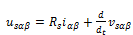(16)

Where,

isαβ = [i, i]T is the stator current vector in the stationary reference frame. Therefore, the desired stator voltage vector can be obtained in a discrete-time form with the information of the incremental flux linkage vector sαβ[k]. Thus,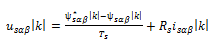(17)

With knowledge of usαβ[k], proper switching signals can be generated by the SVM module to achieve fast and accurate torque and flux linkage control. Since the reference torque angle for the next time interval is directly acquired, the calculated voltage vector can compensate for the torque and flux errors instantaneously. However, the maximum magnitude Vm of the voltage vector that can be provided by the three-phase bridge inverter is Vdc/3, where Vdc is the dc-bus voltage. Once the magnitude of usαβ[k] exceeds Vm, the values of usαβ[k] should be modified as usαβ[k] as follows,(18)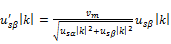(19)

In this way, sinusoidal output voltages can be obtained within the physical limitation of the inverter.

6. Simulations and Results

In order to investigate the effectiveness of the proposed models and control algorithms of the Closed Loop Vector Control and Space Vector PWM of a wind turbine, time-discrete dynamic simulations were implemented using Sim Power Systems of MATLAB/Simulink environment. In order to have a fully functional and stable wind turbine system, both closed loop vector control and space vector PWM are required to operate as they are designed. On top of that, the results of the simulations are analyzed to ensure the output signals are implementable in real condition application. In order to operate a wind turbine system efficiently, grid-side controller is necessary because, the DC-link capacitor voltage varies with the wind. The SVPWM is designed to maintain the DC-link capacitor voltage at a constant magnitude by adjusting the power flow to the electrical grid.

Table 1 shows the range of parameters used to generate the power from wind. From this ranges we made the theoretical calculation of the power output of the generator.

Table 1. PMSG parameters.

 PMSG Ranges No of poles 6 Stator phase resistance Rs 0.425 D-axis Inductances Ld(H) 0.0082 Q-axis Inductances Lq(H) 0.0082 Flux linkage established by magnets 0.433 Inertia 0.01197 Friction factor 0.001189 Rated speed 153 rad/sec Rated current 12 amps Rated power 6 kw

Therefore the power output from the generator using the table 1 is,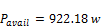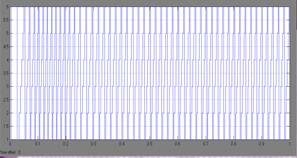Fig. 8. Gate signals of SVPWM.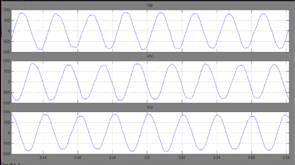Fig. 9. Phase voltage value of inverter output (Va,Vb,Vc).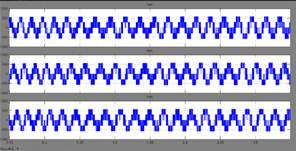Fig. 10. Line voltages value of inverter output (Van,Vbn,Vcn).

Harmonic reduction

PMSG, it is connected to the grid through power electronic converter fully, and even PMSG can omit the difficult gearbox system. Harmonic reduction method can be applied to any of the system to improve power quality . The harmonic component for a typical six pulse rectifier can be calculated from,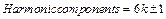(20)

Where k=1, 2, 3…

The harmonic components magnitude will decrease to a very low level when it reaches the 11th harmonic or higher. The 5th and 7thharmonic is the most important harmonics to reduce to a minimum because of their high magnitude. One way to reduce these harmonics is to use an inductor or a low pass filter . Here THD stands for total harmonic distortion and it defines the harmonic distortion in terms of the fundamental current drawn by the load: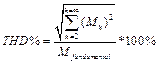(21)

Where, Mh is the magnitude of either the voltage or current harmonic component and Mfundamental is the magnitude of either the fundamental voltage or current.

Figure 11 shows the Harmonic Level or THD Level of the inverter output voltage.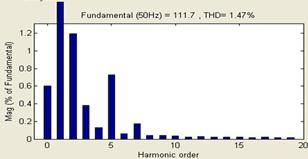Fig. 11. Harmonic Level or THD Level of the inverter output voltage.

7. Conclusion

The paper is developed on the Closed Loop Vector Control and Space Vector PWM of a wind turbine. Direct Drive Permanent Magnet Synchronous Generator is selected due to its high efficiency, high torque-to-size ratio, and low maintenance requirement. Thus the results show that the wind energy conversion using PMSG with SVM-DTC system can achieve excellent performance. And also it has a relatively good quality of output waveform with a low total harmonic distortion values.

References

1. Giuseppe S. Buja, and Marian P. Kazmierkowski, "Direct Torque Control of PWM Inverter-Fed AC Motors—A Survey", IEEE Trans.on Industrial Electronics, Vol. 51, No. 4, August 2004.
2. Md. Enamul Haque, Member, IEEE, Michael Negnevitsky, Senior Member, IEEE, A Novel Control Strategy for a Variable-Speed Wind Turbine With a Permanent-Magnet Synchronous Generator IEEE Trans. on Industry Applications, Vol. 46, No. 1, January/February 2010.
3. Wei Qiao, Member, IEEE, Liyan Qu, Member, IEEE, "Control of IPM Synchronous Generator for Maximum Wind Power Generation Considering Magnetic Saturation",IEEE Trans. on Industry Applications, Vol. 45, No. 3, May/June 2009.
4. S.VIJAYALAKSHMI, "Modeling and control of a Wind Turbine using Permanent Magnet Synchronous Generator", International Journal of Engineering Science and Technology (IJEST).
5. Satyam Kumar Upadhyay, Sudhanshu Tripathi, "Optimization and Control of a Variable Speed Wind Turbine with a Permanent Magnet Synchronous Generator", International Journal of Engineering Trends and Technology (IJETT) - Volume4 Issue6- June 2013.
6. Domenico Casadei, Member, IEEE, Francesco Profumo, Senior Member, IEEE, "FOC and DTC: Two Viable Schemes for Induction Motors Torque Contro", lIEEE Trans. on Power Electronics, Vol. 17, No. 5, September 2002.
7. Anjana Manuel, Jebin Francis, "Simulation of Direct Torque Controlled Induction Motor Drive by using Space Vector Pulse Width Modulation for Torque Ripple Reduction", International l Journal of Advanced Research in Electrical, Electronics and Instrumentation EngineeringVol. 2, Issue 9, September 2013.
8. K.Chikh, A.Saad, M.Khafallah and D.Yousfi," A Novel Drive Implementation for PMSM By using Direct Torque Control with Space Vector Modulation", Canadian Journal on Electrical and Electronics Engineering Vol. 2, No. 8, August 2011.
9. Ackerman T, editor, "Wind Power in Power Systems", West Sussex, England: 2005. Generators and Power Electronics for Wind Turbines; p 53-72.
10. Zhe Zhang, Student Member, IEEE, Yue Zhao, Student Member, IEEE, "A Space-Vector-Modulated Sensor less Direct-Torque Control for Direct-Drive PMSG Wind Turbines", IEEE Trans. on Industry Applications, Vol. 50, No. 4, July/August 2014.
11. Dr. M. Vijaya kumar," Modeling and Control of a Permanent Magnet Synchronous Generator Wind Turbine with Energy Storage ", International Journal of Engineering Research and Applications (IJERA) ISSN: 2248-9622 October 2012, pp.1900-1905.
12. S. Engström, "NewGen a new direct drive wind turbine generator," Nordic Wind PowerConference (NWPC’2006), Hanasaari, Espoo, Finland, 22-23 May 2006.
13. T. Hartkopf, M. Hofmann and S. Jöckel, "Direct-drive generators for megawatt wind turbines", Proc. European Wind Energy Conf., Dublin, Ireland, 1997, pp. 668-671
14. Yicheng Chen, P. Pillay, and A. Khan, "PM wind generator topologies," IEEE Trans. on Ind.Appl., vol. 41, no. 6, pp. 1619- 1626, 2005.
15. Johnson G.L. 2006, "Wind Turbine Power, Energy, and Torque," In Wind Energy System, Electrical Edition ed. Prentice-Hall Englewood Cliffs (NJ), pp. 4-1-4-54.
16. Quang N.P. & Dittrich J.A. 2008, "Inverter control with space vector modulation," In Vector Control of Three-Phase AC Machines, First ed. Springer, pp. 17-59.
17. Espinoza J.R. 2001, "Inverters," In Power Electronics Handbook, Rashid M.H., ed., Oxford University Press, pp. 353-404.
18. Li S.H., Haskew T.A., Swatloski R.P., & Gathings W. 2012," Optimal and Direct-Current Vector Control of Direct-Driven PMSG Wind Turbines", IEEE Transactions on power electronics, 27, (5) 2325-2337.

 Contents 1. 2. 3. 4. 5. 6. 7.
Article ToolsAbstractPDF(1897K)